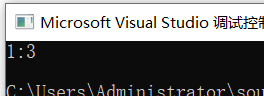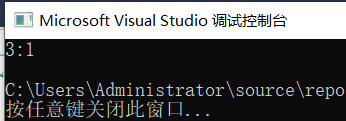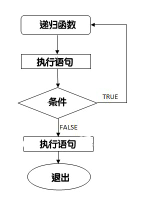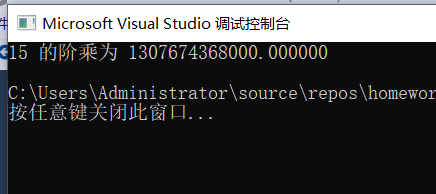# c++函数

## 一、为什么要用函数？

1.使编程模块化，并且可以重复使用提高编程的效率。
2.有利于程序的调试、而且程序的可读性也很好、编程思路更清楚。

#include "pch.h"
#include <iostream>
using namespace std;

// 函数声明
int max(int num1, int num2);

int main ()
{
int a = 1;
int b = 2;
int ret;

// 调用函数来获取最大值
ret = max(a, b);

cout << "Max value is : " << ret << endl;

return 0;
}

// 函数返回两个数中较大的那个数
int max(int num1, int num2)
{
int result;

if (num1 > num2)
result = num1;
else
result = num2;

return result;
}


## 二、为什么使用重载函数？

//定义一个完成求和的函数
//函数声明


//函数声明


## 三、什么是值传递？

#include "pch.h"
#include <iostream>
using namespace std;

void swap(int x, int y) {
int temp = y;
y = x;
x = temp;
}
int main()
{
int x = 1;
int y = 3;

swap(x, y);

cout << x << ":" << y << endl;
return 0;
}## 四、什么是地址传递？


#include "pch.h"
#include <iostream>
using namespace std;

void swap(int *x, int *y) {
int temp = *y;
*y = *x;
*x = temp;
}
int main()
{
int x = 1;
int y = 3;

swap(&x, &y);

cout << x << ":" << y << endl;
return 0;
}## 五、递归函数n!=1×2×3×...×n。阶乘亦可以递归方式定义：0!=1，n!=(n-1)!×n。

#include "pch.h"
#include <iostream>
using namespace std;

double factorial(unsigned int i)
{
if (i <= 1)
{
return 1;
}
return i * factorial(i - 1);
}
int  main()
{
int i = 15;
printf("%d 的阶乘为 %f\n", i, factorial(i));
return 0;
}posted @ 2019-09-14 21:20  罗斯柴尔德  阅读(241)  评论(0编辑  收藏  举报